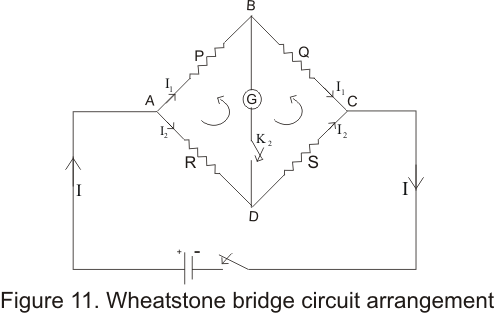# Wheat stone bridge

## Wheat stone bridge

• Wheat stone bridge was designed by British physicist sir Charles F Wheatstone in 1833
• It is a arrangement of four resistors used to determine resistance of one resistors in terms of other three resistors
• Consider the figure given below which is an arrangement of resistors and is knowns as wheat stone bridge• Wheatstone bridge consists of four resistance P,Q,R and S with a battery of EMF E.Two keys K1 and K2 are connected across terminals A and C and B and D respectively
• ON pressing key K1 first and then pressing K2 next if galvanometer does not show any deflection then Wheatstone bridge is said to be balanced
• Galvanometer is not showing any deflection this means that no current is flowing through the galvanometer and terminal B and D are at the same
potential .THus for a balanced bridge
VB=VD
• Now we have to find the condition for the balanced Wheatstone bridge .For this applying Kirchhoff's loop rule to the loop ABDA ,we find the relation
-I2R+I1P=0
or I1P=I2R --(a)
Again applying Kirchhoff's rule to the loop BCDB
I1Q-I2S=0
or I1Q=I2S --(b)
From equation a and b we get
I1/I2=R/P=S/Q
or
P/Q=R/S                       (12)
• equation 12 gives the condition for the balanced Wheatstone bridge
• Thus if the ratio of the resistance R is known then unknown resistance S can easily be calculated
• One important thing to note is that when bridge is balanced positions of cell and galvanometer can be exchanged without having any effect on the balance of the bridge
• Sensitivity of the bridge depends on the relative magnitudes of the resistance in the four arm of the bridge is maximum for same order of four resistance.